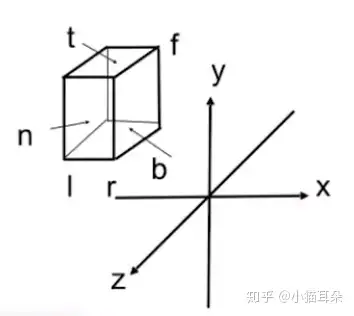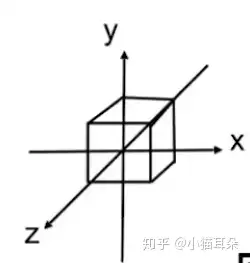# 有趣。# Perspective

You should have known the homogeneous coordinates

Here we just provide a simple affine projection matrix, first:

## 3D affine transformations

note: the $c$ is a constant number.

## Perspective Projection Derivation

### Orthographic projectionFirst of all, move the object’s center onto origin $(0,0,0)$ .

Then, normalize the object.

And multiply them:

### Step into the deep.Through observing the figure, we see that there is a similar triangle
and then:

Similarly:

Then we have a mapping matrix:

Here, we need know some rules:

1. Points of the near plane are constant.

2. The coordinates $(0, 0, f, 1)$ of the centric point of the far plane are unchanged.

3. The values of points’ $z$ coordinate on the far plane are always $f$.

Because of rule 3, we can get a equaltion:

Suppose the thrid row of the above matrix are $(A, B, C, D)$ .

And, it’s clear that

Then we could get:

According the rule 2, the coordinates of the centric point of the
far plane are $(0, 0, f, 1)$ .

Take it in the above matrix and get the following matrix;

Like the above equaltion, get the following equaltion:

There are two equaltions with two varibles.

Finally, we get the perspective projection matrix:

## Rodrigoues’ Rotation Formula

Rotation by angle $\alpha$ around axis $n$ .

GAMES101: P4 lecture Transformation Cont.

GAMES101: 现代计算机图形学入门

Perspective Projection Derivation